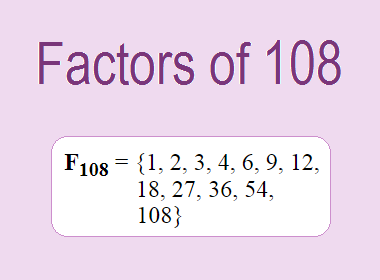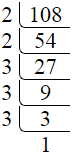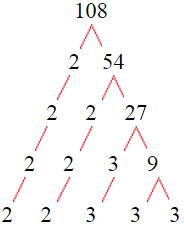# Factors of 108The factors of 108 are 1, 2, 3, 4, 6, 9, 12, 18, 27, 36, 54, and 108 i.e. F108 = {1, 2, 3, 4, 6, 9, 12, 18, 27, 36, 54, 108}. The factors of 108 are those numbers that can divide 108 without leaving a remainder.

We can check if these numbers are factors of 108 by dividing 108 by each of them. If the result is a whole number, then the number is a factor of 108. Let's do this for each of the numbers listed above:

·        1 is a factor of 108 because 108 divided by 1 is 108.

·        2 is a factor of 108 because 108 divided by 2 is 54.

·        3 is a factor of 108 because 108 divided by 3 is 36.

·        4 is a factor of 108 because 108 divided by 4 is 27.

·        6 is a factor of 108 because 108 divided by 6 is 18.

·        9 is a factor of 108 because 108 divided by 9 is 12.

·        12 is a factor of 108 because 108 divided by 12 is 9.

·        18 is a factor of 108 because 108 divided by 18 is 6.

·        27 is a factor of 108 because 108 divided by 27 is 4.

·        36 is a factor of 108 because 108 divided by 36 is 3.

·        54 is a factor of 108 because 108 divided by 54 is 2.

·        108 is a factor of 108 because 108 divided by 108 is 1.

## How to Find Factors of 108?

1 and the number itself are the factors of every number. So, 1 and 108 are two factors of 108. To find the other factors of 108, we can start by dividing 108 by the numbers between 1 and 108. If we divide 108 by 2, we get a remainder of 0. Therefore, 2 is a factor of 108. If we divide 108 by 3, we get a remainder of 0. Therefore, 3 is also a factor of 108.

Next, we can check if 4 is a factor of 108. If we divide 108 by 4, we get a remainder of 0. Therefore, 4 is also a factor of 108. If we divide 108 by 5, we get a remainder of 3. Therefore, 5 is not a factor of 108. We can continue this process for all the possible factors of 108.

Through this process, we can find that the factors of 108 are 1, 2, 3, 4, 6, 9, 12, 18, 27, 36, 54, and 108. These are the only numbers that can divide 108 without leaving a remainder.

********************

********************

## Properties of the Factors of 108

The factors of 108 have some interesting properties. One of the properties is that the sum of the factors of 108 is equal to 280. We can see this by adding all the factors of 108 together:

1 + 2 + 3 + 4 + 6 + 9 + 12 + 18 + 27 + 36 + 54 + 108 = 280

Another property of the factors of 108 is that the prime factors of 108 are 2, and 3 only and there are all composite numbers except 1, 2, and 3.

## Applications of the Factors of 108

The factors of 108 have several applications in mathematics. One of the applications is in finding the highest common factor (HCF) of two or more numbers. The HCF is the largest factor that two or more numbers have in common. For example, to find the HCF of 108 and 120, we need to find the factors of both numbers and identify the largest factor they have in common. The factors of 108 are 1, 2, 3, 4, 6, 9, 12, 18, 27, 36, 54, and 108. The factors of 120 are 1, 2, 3, 5, 6, 8, 10, 12, 15, 20, 24, 30, 40, 60, and 120. The largest factor that they have in common is 12. Therefore, the HCF of 108 and 120 is 12.

Another application of the factors of 108 is in prime factorization. Prime factorization is the process of expressing a number as the product of its prime factors. The prime factors of 108 are 2, and 3, since these are the only prime numbers that can divide 108 without leaving a remainder. Therefore, we can express 108 as:

108 = 2 × 2 × 3 × 3 × 3

We can do prime factorization by division and factor tree method also. Here is the prime factorization of 108 by division method,108 = 2 × 2 × 3 × 3 × 3

Here is the prime factorization of 108 by the factor tree method,108 = 2 × 2 × 3 × 3 × 3## Conclusion

The factors of 108 are the numbers that can divide 108 without leaving a remainder. The factors of 108 are 1, 2, 3, 4, 6, 9, 12, 18, 27, 36, 54, and 108. The factors of 108 have some interesting properties, such as having a sum of 280. The factors of 108 have several applications in mathematics, such as finding the highest common factor and prime factorization.